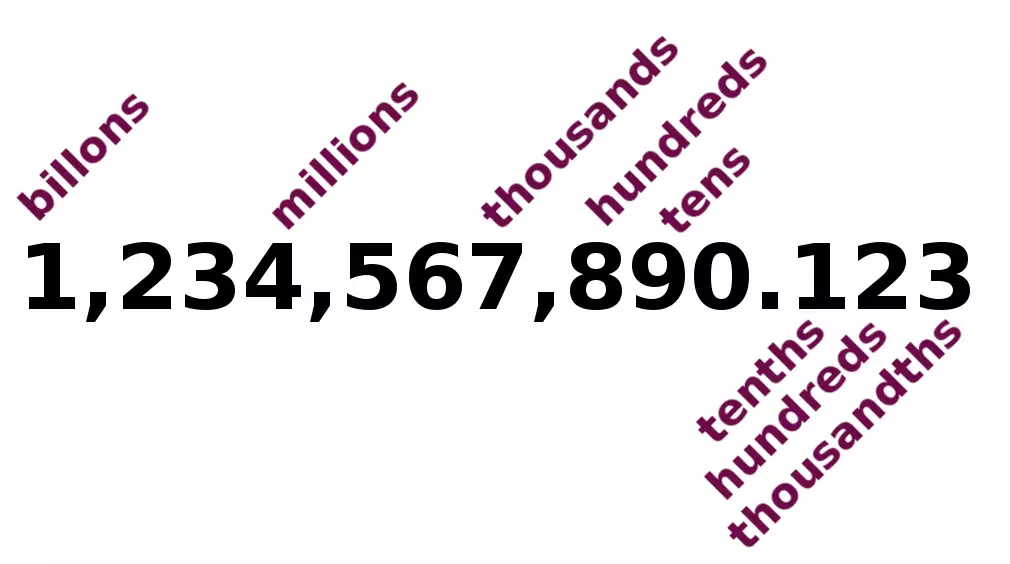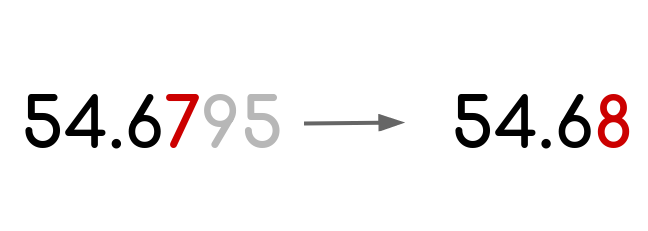# Rounding calculatorRounding values to the nearest significant figure may seem like a simple task. In many cases, it is. Approximating a fractional decimal to the nearest natural number is simple, even for kids.

If you try rounding up 8957303479453 to the nearest billion, then using our tool will be helpful.

### Rounding whole numbers

This tool will help you round off whole numbers to the nearest hundreds, thousands, millions, or billions. Simply pick a significant digit in a "Round to" field and click "Calculate".

You can put positive or negative numbers into our tool.

### Rounding decimalsOur rounding decimals calculator will not only allow you to set rounding precision but let you set your preferred approximation method:

• Rounding up
• Rounding down
• Rounding towards zero
• Rounding away from zero
• Rounding mixed numbers

This type of computation may be tricky, but you can simply convert a mixed number to decimal and round it using the form above.

A useful calculator for rounding decimals to the nearest whole numbers, tenth, hundredth, or a thousandth.

If you deal with fractions, you may use our rounding fractions calculator. It will not only convert a fraction to decimal but will round it to the nearest decimal with a chosen number of significant digits.

## Rounding Methods

There are many different rounding modes, and you should be aware of them to properly choose your approximation technique.

### Half up

This is the most common form of approximating values and how you were used to rounding at school. This method rounds the decimal number to the nearest natural number. If the fraction part is equal to or greater than .5, then the value goes up. Otherwise, the value goes down. Let’s see examples:

• 1,5 => 2
• 1,51 => 2
• 1,49 => 1

### Half down

This is a close variation of the previous method. The only difference is that the fraction part must be greater than .5 in order to be able to increase the value. Check the examples:

• 1,5 => 1 (opposite behavior)
• 1,51 => 2
• 1,49 => 1

### Round up (ceiling)

This is a very convenient method when you want to approximate a decimal to the next closest natural number.

• 1,5 => 2
• 1,51 => 2
• 1,49 => 2
• 1,01 => 2

### Round down (floor)

There is an opposite method to the previous one. When you want to approximate decimal to the previous closest natural number, in other words, you simply want to cut off fractional parts by removing numbers after the decimal point.

• 1,99 => 1
• 1,51 => 1
• 1,49 => 1
• 1,01 => 1

### Round half to even

This is the less common method when the value is approximated not to the nearest integer but to the nearest even number. Let’s see some examples:

• 1,99 => 2
• 2,51 => 4
• 2,49 => 2
• 3,49 => 2

### Round half to odd

Very similar to the rule described before, with a distinction that values are rounded to the nearest odd integer.

• 1,99 => 3
• 2,51 => 3
• 2,49 => 1
• 3,49 => 3

This and the previous procedure are also known as tie-breaking rules.

### Round half toward zeros

This method approximates positive and negative symmetrically, with zero as a bias. For example, 2.1 gets rounded to 2, and -2.1 is rounded to -2.

This method is also called round half away from infinity.

### Round half away from zero.

This is the opposite behavior of the previous one. The positive and negative numbers are rounded symmetrically, with zero as a bias. This method is also called round half toward infinity. For a given number:

• 2.1 => 3
• - 2.1 => -3

You may be surprised by the fact that it is a very popular method for rounding prices and currency conversion. Thus, it’s also known as commercial rounding.

## Sig Fig Rounding Calculator

Rounding to the nearest significant figure is useful in real life, as in some situations, we care about the most important figures when comparing numbers.

For example, when comparing maximum ship payload, we care about the number of tons, not kilograms. Therefore you need to round to max ship capacity to the nearest thousand.

• Round to the Nearest Whole
• Round to the Nearest Ten
• Round to the Nearest Hundred
• Round to the Nearest Thousand
• Round to the Nearest Million

### Digits after decimal pointOften not all decimal places are important. In order to simplify the comparison, it is common practice to just cut off not necessary digits after the comma.

The most common cases are:

• Round to the Nearest Tenth
• Round to the Nearest Hundredth
• Round to the Nearest Thousandth

The plentifulness of use cases makes it one most common methods, not only for approximating traditional money amounts but for cryptocurrencies as well, where even digits to the right of hundred-thousandths are important. For more details visit our rounding money calculator.

## Examples

### Round to the nearest ten

Convert the decimal number to the nearest tens: 43543.67434 => 43540

### Round to the nearest whole number

Convert the decimal number to the nearest integer: 43543.67434 => 43544

### Round to the nearest tenth

Convert the decimal number to the nearest integer: 43543.67434 => 43543.7

### Round to the nearest hundredth

Convert the decimal number to the nearest integer: 43543.67434 => 43543.67

## Summary

Now that you are familiar with different ways of comparing numbers, you can select the desired rounding procedure in our free online tool and perform your own calculations.

This may seem like a boring topic, but understanding it will prevent you from making many mistakes when comparing numbers and help you with data analysis. If you need to dive into this topic, we recommend you read this Wikipedia article.

### Authors

Created by Lucas Krysiak on 2022-03-21 16:53:53 | Last review by Mike Kozminsky on 2022-09-17 16:44:48